$$\newcommand{\id}{\mathrm{id}}$$ $$\newcommand{\Span}{\mathrm{span}}$$ $$\newcommand{\kernel}{\mathrm{null}\,}$$ $$\newcommand{\range}{\mathrm{range}\,}$$ $$\newcommand{\RealPart}{\mathrm{Re}}$$ $$\newcommand{\ImaginaryPart}{\mathrm{Im}}$$ $$\newcommand{\Argument}{\mathrm{Arg}}$$ $$\newcommand{\norm}{\| #1 \|}$$ $$\newcommand{\inner}{\langle #1, #2 \rangle}$$ $$\newcommand{\Span}{\mathrm{span}}$$

# 7.4: Factor Special Products

•• OpenStax
• OpenStax
$$\newcommand{\vecs}{\overset { \rightharpoonup} {\mathbf{#1}} }$$ $$\newcommand{\vecd}{\overset{-\!-\!\rightharpoonup}{\vphantom{a}\smash {#1}}}$$$$\newcommand{\id}{\mathrm{id}}$$ $$\newcommand{\Span}{\mathrm{span}}$$ $$\newcommand{\kernel}{\mathrm{null}\,}$$ $$\newcommand{\range}{\mathrm{range}\,}$$ $$\newcommand{\RealPart}{\mathrm{Re}}$$ $$\newcommand{\ImaginaryPart}{\mathrm{Im}}$$ $$\newcommand{\Argument}{\mathrm{Arg}}$$ $$\newcommand{\norm}{\| #1 \|}$$ $$\newcommand{\inner}{\langle #1, #2 \rangle}$$ $$\newcommand{\Span}{\mathrm{span}}$$ $$\newcommand{\id}{\mathrm{id}}$$ $$\newcommand{\Span}{\mathrm{span}}$$ $$\newcommand{\kernel}{\mathrm{null}\,}$$ $$\newcommand{\range}{\mathrm{range}\,}$$ $$\newcommand{\RealPart}{\mathrm{Re}}$$ $$\newcommand{\ImaginaryPart}{\mathrm{Im}}$$ $$\newcommand{\Argument}{\mathrm{Arg}}$$ $$\newcommand{\norm}{\| #1 \|}$$ $$\newcommand{\inner}{\langle #1, #2 \rangle}$$ $$\newcommand{\Span}{\mathrm{span}}$$

##### Learning Objectives

By the end of this section, you will be able to:

• Factor perfect square trinomials
• Factor differences of squares
• Factor sums and differences of cubes
• Choose method to factor a polynomial completely
##### Note

Before you get started, take this readiness quiz.

1. Simplify: $$(12 x)^{2}$$
If you missed this problem, review Exercise 6.2.22.
2. Multiply: $$(m+4)^{2}$$
If you missed this problem, review Exercise 6.4.1.
3. Multiply: $$(p-9)^{2}$$
If you missed this problem, review Exercise 6.4.4.
4. Multiply: $$(k+3)(k-3)$$
If you missed this problem, review Exercise 6.4.16.

The strategy for factoring we developed in the last section will guide you as you factor most binomials, trinomials, and polynomials with more than three terms. We have seen that some binomials and trinomials result from special products—squaring binomials and multiplying conjugates. If you learn to recognize these kinds of polynomials, you can use the special products patterns to factor them much more quickly.

## Factor Perfect Square Trinomials

Some trinomials are perfect squares. They result from multiplying a binomial times itself. You can square a binomial by using FOIL, but using the Binomial Squares pattern you saw in a previous chapter saves you a step. Let’s review the Binomial Squares pattern by squaring a binomial using FOIL.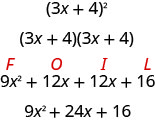The first term is the square of the first term of the binomial and the last term is the square of the last. The middle term is twice the product of the two terms of the binomial.

$\begin{array}{c}{(3 x)^{2}+2(3 x \cdot 4)+4^{2}} \\ {9 x^{2}+24 x+16}\end{array}$

The trinomial $$9 x^{2}+24+16$$ is called a perfect square trinomial. It is the square of the binomial 3x+4.

We’ll repeat the Binomial Squares Pattern here to use as a reference in factoring.

##### BINOMIAL SQUARES PATTERN

If a and b are real numbers,

$(a+b)^{2}=a^{2}+2 a b+b^{2} \qquad(a-b)^{2}=a^{2}-2 a b+b^{2}$

When you square a binomial, the product is a perfect square trinomial. In this chapter, you are learning to factor—now, you will start with a perfect square trinomial and factor it into its prime factors.

You could factor this trinomial using the methods described in the last section, since it is of the form $$ax^{2}+bx+c$$. But if you recognize that the first and last terms are squares and the trinomial fits the perfect square trinomials pattern, you will save yourself a lot of work.

Here is the pattern—the reverse of the binomial squares pattern.

##### PERFECT SQUARE TRINOMIALS PATTERN

If a and b are real numbers,

$a^{2}+2 a b+b^{2}=(a+b)^{2} \qquad a^{2}-2 a b+b^{2}=(a-b)^{2}$

To make use of this pattern, you have to recognize that a given trinomial fits it. Check first to see if the leading coefficient is a perfect square, $$a^2$$. Next check that the last term is a perfect square, $$b^2$$. Then check the middle term—is it twice the product, $$2ab$$? If everything checks, you can easily write the factors.

##### Exercise $$\PageIndex{1}$$: How to Factor Perfect Square Trinomials

Factor: $$9 x^{2}+12 x+4$$##### Exercise $$\PageIndex{2}$$

Factor: $$4 x^{2}+12 x+9$$

$$(2 x+3)^{2}$$

##### Exercise $$\PageIndex{3}$$

Factor: $$9 y^{2}+24 y+16$$

$$(3 y+4)^{2}$$

The sign of the middle term determines which pattern we will use. When the middle term is negative, we use the pattern $$a^{2}-2 a b+b^{2}$$, which factors to $$(a-b)^{2}$$.

The steps are summarized here.

##### FACTOR PERFECT SQUARE TRINOMIALS.

$$\begin{array} {lcc} \textbf { Step 1} \text { . Does the trinomial fit the pattern? } & a^{2}+2 a b+b^{2} & a^{2}-2 a b+b^{2} \\ \qquad \bullet \text { Is the first term a perfect square? } & (a)^{2} & (a)^{2} \\ \qquad \quad\text { Write it as a square. } \\ \qquad \bullet \text { Is the last term a perfect square? } & (a)^{2} \qquad\quad (b)^{2} & (a)^{2} \qquad \quad(b)^{2} \\ \qquad \quad \text { Write it as a square. } \\ \qquad \bullet \text { Check the middle term. Is it } 2 a b ? & (a)^{2} \searrow_{2 \cdot a \cdot b }\swarrow(b)^{2} & (a)^{2} \searrow_{2 \cdot a \cdot b} \swarrow(b)^{2} \\ \textbf { Step 2} . \text { Write the square of the binomial. } & (a+b)^{2} & (a-b)^{2} \\ \textbf { Step 3} . \text { Check by multiplying. }\end{array}$$

We’ll work one now where the middle term is negative.

##### Exercise $$\PageIndex{4}$$

Factor: $$81 y^{2}-72 y+16$$

The first and last terms are squares. See if the middle term fits the pattern of a perfect square trinomial. The middle term is negative, so the binomial square would be $$(a-b)^{2}$$.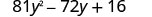Are the first and last terms perfect squares?Check the middle term.Does is match $$(a-b)^{2}$$? Yes.Write the square of a binomial.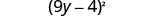Check by multiplying. $$(9 y-4)^{2}$$ $$(9 y)^{2}-2 \cdot 9 y \cdot 4+4^{2}$$ $$81 y^{2}-72 y+16 \checkmark$$
##### Exercise $$\PageIndex{5}$$

Factor: $$64 y^{2}-80 y+25$$

$$(8 y-5)^{2}$$

##### Exercise $$\PageIndex{6}$$

Factor: $$16 z^{2}-72 z+81$$

$$(4 z-9)^{2}$$

The next example will be a perfect square trinomial with two variables.

##### Exercise $$\PageIndex{7}$$

Factor: $$36 x^{2}+84 x y+49 y^{2}$$Test each term to verify the pattern.Factor.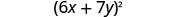Check by multiplying. $$(6 x+7 y)^{2}$$ $$(6 x)^{2}+2 \cdot 6 x \cdot 7 y+(7 y)^{2}$$ $$36 x^{2}+84 x y+49 y^{2} \checkmark$$
##### Exercise $$\PageIndex{8}$$

Factor: $$49 x^{2}+84 x y+36 y^{2}$$

$$(7 x+6 y)^{2}$$

##### Exercise $$\PageIndex{9}$$

Factor: $$64 m^{2}+112 m n+49 n^{2}$$

$$(8 m+7 n)^{2}$$

##### Exercise $$\PageIndex{10}$$

Factor: $$9 x^{2}+50 x+25$$

$$\begin{array}{lc} & 9 x^{2}+50 x+25 \\ \text { Are the first and last terms perfect squares? } & (3 x)^{2} \qquad\quad (5)^2 \\ \text { Check the middle term-is it 2ab? } & (3 x)^{2} \searrow_{2(3 x)(5) }\swarrow (5)^{2}. \\ & \tiny{30x} \\ \text { No! } 30 x \neq 50 x & \text { This does not fit the pattern! } \\ \text { Factor using the "ac" method. } & 9 x^{2}+50 x+25 \\ \begin{array}{c}{\text { ac }} \\ {\text { Notice: } 9 \cdot 25 \text { and } 5 \cdot 45=225} \\ {225}\end{array} \\ {\text { Split the middle term. }} & \begin{array}{c}{9 x^{2}+5 x+45 x+25} \\ {x(9 x+5)+5(9 x+5)} \\ {(9 x+5)(x+5)}\end{array}\\ {\text { Factor by grouping. }} \\ \text { Check. } & \\ \begin{array}{l}{(9 x+5)(x+5)} \\ {9 x^{2}+45 x+5 x+25} \\ {9 x^{2}+50 x+25}\checkmark\end{array}\end{array}$$

##### Exercise $$\PageIndex{11}$$

Factor: $$16 r^{2}+30 r s+9 s^{2}$$

$$(8 r+3 s)(2 r+3 s)$$

##### Exercise $$\PageIndex{12}$$

Factor: $$9 u^{2}+87 u+100$$

$$(3 u+4)(3 u+25)$$

Remember the very first step in our Strategy for Factoring Polynomials? It was to ask “is there a greatest common factor?” and, if there was, you factor the GCF before going any further. Perfect square trinomials may have a GCF in all three terms and it should be factored out first. And, sometimes, once the GCF has been factored, you will recognize a perfect square trinomial.

##### Exercise $$\PageIndex{13}$$

Factor: $$36 x^{2} y-48 x y+16 y$$

 $$36 x^{2} y-48 x y+16 y$$ Is there a GCF? Yes, 4y, so factor it out. 4$$y\left(9 x^{2}-12 x+4\right)$$ Is this a perfect square trinomial? Verify the pattern.Factor. 4$$y(3 x-2)^{2}$$ Remember: Keep the factor 4y in the final product. Check. $$4y(3 x-2)^{2}$$ $$4y[(3 x)^{2}-2 \cdot 3 x \cdot 2+2^{2}]$$ $$4 y(9 x)^{2}-12 x+4$$ $$36 x^{2} y-48 x y+16 y\checkmark$$
##### Exercise $$\PageIndex{14}$$

Factor: $$8 x^{2} y-24 x y+18 y$$

2$$y(2 x-3)^{2}$$

##### Exercise $$\PageIndex{15}$$

Factor: $$27 p^{2} q+90 p q+75 q$$

3$$q(3 p+5)^{2}$$

## Factor Differences of Squares

The other special product you saw in the previous was the Product of Conjugates pattern. You used this to multiply two binomials that were conjugates. Here’s an example:

$\begin{array}{c}{(3 x-4)(3 x+4)} \\ {9 x^{2}-16}\end{array}$

Remember, when you multiply conjugate binomials, the middle terms of the product add to 0. All you have left is a binomial, the difference of squares.

Multiplying conjugates is the only way to get a binomial from the product of two binomials.

##### PRODUCT OF CONJUGATES PATTERN

If a and b are real numbers

$(a-b)(a+b)=a^{2}-b^{2}$

The product is called a difference of squares.

To factor, we will use the product pattern “in reverse” to factor the difference of squares. A difference of squares factors to a product of conjugates.

##### DIFFERENCE OF SQUARES PATTERN

If a and b are real numbers,Remember, “difference” refers to subtraction. So, to use this pattern you must make sure you have a binomial in which two squares are being subtracted.

##### Exercise $$\PageIndex{16}$$: How to Factor Differences of Squares

Factor: $$x^{2}-4$$##### Exercise $$\PageIndex{17}$$

Factor: $$h^{2}-81$$

$$(h-9)(h+9)$$

##### Exercise $$\PageIndex{18}$$

Factor: $$k^{2}-121$$

$$(k-11)(k+11)$$

##### FACTOR DIFFERENCES OF SQUARES.

$$\begin{array}{lc} \textbf { Step 1} . \text { Does the binomial fit the pattern? } & a^{2}-b^{2} \\ \qquad \bullet \text { Is this a difference? } & \underline{\quad} - \underline{\quad} \\ \qquad \bullet \text { Are the first and last terms perfect squares? } \\ \textbf { Step 2} . \text { Write them as squares. } & (a)^{2}-(b)^{2} \\ \textbf { Step 3.} \text{ Write the product of conjugates. } & (a-b)(a+b) \\ \textbf { Step 4.} \text{ Check by multiplying. } \end{array}$$

It is important to remember that sums of squares do not factor into a product of binomials. There are no binomial factors that multiply together to get a sum of squares. After removing any GCF, the expression $$a^{2}+b^{2}$$ is prime!

Don’t forget that 1 is a perfect square. We’ll need to use that fact in the next example.

##### Exercise $$\PageIndex{19}$$

Factor: $$64 y^{2}-1$$Is this a difference? Yes.Are the first and last terms perfect squares? Yes - write them as squares.Factor as the product of conjugates.Check by multiplying. $$(8 y-1)(8 y+1)$$ $$64 y^{2}-1 \checkmark$$
##### Exercise $$\PageIndex{20}$$

Factor: $$m^{2}-1$$

$$(m-1)(m+1)$$

##### Exercise $$\PageIndex{21}$$

Factor: $$81 y^{2}-1$$

$$(9 y-1)(9 y+1)$$

##### Exercise $$\PageIndex{22}$$

Factor: $$121 x^{2}-49 y^{2}$$

$$\begin{array}{lc} & 121 x^{2}-49 y^{2} \\ \text { Is this a difference of squares? Yes. } & (11 x)^{2}-(7 y)^{2} \\ \text { Factor as the product of conjugates. } & (11 x-7 y)(11 x+7 y) \\ \text { Check by multiplying. } & \\ \begin{array}{l}{(11 x-7 y)(11 x+7 y)} \\ {121 x^{2}-49 y^{2}} \checkmark \end{array} \end{array}$$

##### Exercise $$\PageIndex{23}$$

Factor: $$196 m^{2}-25 n^{2}$$

$$(16 m-5 n)(16 m+5 n)$$

##### Exercise $$\PageIndex{24}$$

Factor: $$144 p^{2}-9 q^{2}$$

$$(12 p-3 q)(12 p+3 q)$$

The binomial in the next example may look “backwards,” but it’s still the difference of squares.

##### Exercise $$\PageIndex{25}$$

Factor: $$100-h^{2}$$

$$\begin{array}{lc} & 100-h^{2} \\ \text { Is this a difference of squares? Yes. } & (10)^{2}-(h)^{2}\\ \text { Factor as the product of conjugates. } & (10-h)(10+h)\\ \text { Check by multiplying. } & \\ \begin{array}{l}{(10-h)(10+h)} \\ {100-h^{2}} \checkmark \end{array} \end{array}$$

Be careful not to rewrite the original expression as $$h^{2}-100$$.

Factor $$h^{2}-100$$ on your own and then notice how the result differs from $$(10-h)(10+h)$$.

##### Exercise $$\PageIndex{26}$$

Factor: $$144-x^{2}$$

$$(12-x)(12+x)$$

##### Exercise $$\PageIndex{27}$$

Factor: $$169-p^{2}$$

$$(13-p)(13+p)$$

To completely factor the binomial in the next example, we’ll factor a difference of squares twice!

##### Exercise $$\PageIndex{28}$$

Factor: $$x^{4}-y^{4}$$

$$\begin{array}{lc}\text { Is this a difference of squares? Yes. } & {x^{4}-y^{4}} \\\text { Factor it as the product of conjugates. } & {\left(x^{2}\right)^{2}-\left(y^{2}\right)^{2}} \\ \text { Notice the first binomial is also a difference of squares! } & {\left(x^{2}-y^{2}\right)\left(x^{2}+y^{2}\right)} \\ \text { Factor it as the product of conjugates. The last }& {(x-y)(x+y)\left(x^{2}+y^{2}\right)} \\ \text { factor, the sum of squares, cannot be factored. } \\ \\ \text { Check by multiplying. } & \\\begin{array}{l}{(x-y)(x+y)\left(x^{2}+y^{2}\right)} \\ {[(x-y)(x+y)]\left(x^{2}+y^{2}\right)} \\ {\left(x^{2}-y^{2}\right)\left(x^{2}+y^{2}\right)} \\ {x^{4}-y^{4}} \checkmark \end{array} \end{array}$$

##### Exercise $$\PageIndex{29}$$

Factor: $$a^{4}-b^{4}$$

$$\left(a^{2}+b^{2}\right)(a+b)(a-b)$$

##### Exercise $$\PageIndex{30}$$

Factor: $$x^{4}-16$$

$$\left(x^{2}+4\right)(x+2)(x-2)$$

As always, you should look for a common factor first whenever you have an expression to factor. Sometimes a common factor may “disguise” the difference of squares and you won’t recognize the perfect squares until you factor the GCF.

##### Exercise $$\PageIndex{31}$$

Factor: $$8 x^{2} y-98 y$$

$$\begin{array}{lc}& 8 x^{2} y-98 y \\ \text { Is there a GCF? Yes, } 2 y-\text { factor it out! } & 2 y\left(4 x^{2}-49\right) \\ \text { Is the binomial a difference of squares? Yes. } & 2 y\left((2 x)^{2}-(7)^{2}\right) \\ \text { Factor as a product of conjugates. } & 2 y(2 x-7)(2 x+7) \\ \text { Check by multiplying. } \\ \\ \begin{array}{l}{2 y(2 x-7)(2 x+7)} \\ {2 y[(2 x-7)(2 x+7)]} \\ {2 y\left(4 x^{2}-49\right)} \\ {8 x^{2} y-98 y} \checkmark \end{array} \end{array}$$

##### Exercise $$\PageIndex{31}$$

Factor: $$7 x y^{2}-175 x$$

7$$x(y-5)(y+5)$$

##### Exercise $$\PageIndex{32}$$

Factor: $$45 a^{2} b-80 b$$

5$$b(3 a-4)(3 a+4)$$

##### Exercise $$\PageIndex{33}$$

Factor: $$6 x^{2}+96$$

$$\begin{array}{lc}&6 x^{2}+96 \\ \text { Is there a GCF? Yes, } 6-\text { factor it out! } & 6\left(x^{2}+16\right) \\ \text { Is the binomial a difference of squares? No, it } & \\ \text { is a sum of squares. Sums of squares do not factor! } & \\ \text { Check by multiplying. } \\ \\ \begin{array}{l}{6\left(x^{2}+16\right)} \\ {6 x^{2}+96 }\checkmark \end{array} \end{array}$$

##### Exercise $$\PageIndex{34}$$

Factor: $$8 a^{2}+200$$

8$$\left(a^{2}+25\right)$$

##### Exercise $$\PageIndex{35}$$

Factor: $$36 y^{2}+81$$

9$$\left(4 y^{2}+9\right)$$

## Factor Sums and Differences of Cubes

There is another special pattern for factoring, one that we did not use when we multiplied polynomials. This is the pattern for the sum and difference of cubes. We will write these formulas first and then check them by multiplication.

\begin{aligned} a^{3}+b^{3} &=(a+b)\left(a^{2}-a b+b^{2}\right) \\ a^{3}-b^{3} &=(a-b)\left(a^{2}+a b+b^{2}\right) \end{aligned}

We’ll check the first pattern and leave the second to you.Distribute.Multiply. $$a^{3}-a^{2} b+a b^{2}+a^{2} b-a b^{2}+b^{3}$$ Combine like terms. $$a^{3}+b^{3}$$
##### SUM AND DIFFERENCE OF CUBES PATTERN

$\begin{array}{l}{a^{3}+b^{3}=(a+b)\left(a^{2}-a b+b^{2}\right)} \\ {a^{3}-b^{3}=(a-b)\left(a^{2}+a b+b^{2}\right)}\end{array}$

The two patterns look very similar, don’t they? But notice the signs in the factors. The sign of the binomial factor matches the sign in the original binomial. And the sign of the middle term of the trinomial factor is the opposite of the sign in the original binomial. If you recognize the pattern of the signs, it may help you memorize the patterns.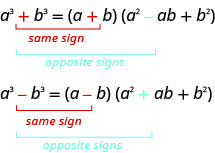The trinomial factor in the sum and difference of cubes pattern cannot be factored.

It can be very helpful if you learn to recognize the cubes of the integers from 1 to 10, just like you have learned to recognize squares. We have listed the cubes of the integers from 1 to 10 in Figure $$\PageIndex{1}$$.Figure $$\PageIndex{1}$$
##### Exercise $$\PageIndex{36}$$: How to Factor the Sum or Difference of Cubes

Factor: $$x^{3}+64$$##### Exercise $$\PageIndex{37}$$

Factor: $$x^{3}+27$$

$$(x+3)\left(x^{2}-3 x+9\right)$$

##### Exercise $$\PageIndex{38}$$

Factor: $$y^{3}+8$$

$$(y+2)\left(y^{2}-2 y+4\right)$$

##### FACTOR THE SUM OR DIFFERENCE OF CUBES.

To factor the sum or difference of cubes:

1. Does the binomial fit the sum or difference of cubes pattern?
• Is it a sum or difference?
• Are the first and last terms perfect cubes?
2. Write them as cubes.
3. Use either the sum or difference of cubes pattern.
4. Simplify inside the parentheses
5. Check by multiplying the factors.
##### Exercise $$\PageIndex{39}$$

Factor: $$: x^{3}-1000$$This binomial is a difference. The first and last terms are perfect cubes. Write the terms as cubes.Use the difference of cubes pattern.Simplify.Check by multiplying.##### Exercise $$\PageIndex{40}$$

Factor: $$u^{3}-125$$

$$(u-5)\left(u^{2}+5 u+25\right)$$

##### Exercise $$\PageIndex{41}$$

Factor: $$v^{3}-343$$

$$(v-7)\left(v^{2}+7 v+49\right)$$

Be careful to use the correct signs in the factors of the sum and difference of cubes.

##### Exercise $$\PageIndex{42}$$

Factor: $$512-125 p^{3}$$This binomial is a difference. The first and last terms are perfect cubes. Write the terms as cubes.Use the difference of cubes pattern.Simplify.Check by multiplying. We'll leave the check to you.
##### Exercise $$\PageIndex{43}$$

Factor: $$64-27 x^{3}$$

$$(4-3 x)\left(16+12 x+9 x^{2}\right)$$

##### Exercise $$\PageIndex{44}$$

Factor: $$27-8 y^{3}$$

$$(3-2 y)\left(9+6 y+4 y^{2}\right)$$

##### Exercise $$\PageIndex{45}$$

Factor: $$27 u^{3}-125 v^{3}$$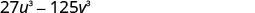This binomial is a difference. The first and last terms are perfect cubes. Write the terms as cubes.Use the difference of cubes pattern.Simplify.Check by multiplying. We'll leave the check to you.
##### Exercise $$\PageIndex{46}$$

Factor: $$8 x^{3}-27 y^{3}$$

$$(2 x-3 y)\left(4 x^{2}+6 x y+9 y^{2}\right)$$

##### Exercise $$\PageIndex{47}$$

Factor: $$1000 m^{3}-125 n^{3}$$

$$(10 m-5 n)\left(100 m^{2}+50 m n+25 n^{2}\right)$$

In the next example, we first factor out the GCF. Then we can recognize the sum of cubes.

##### Exercise $$\PageIndex{48}$$

Factor: $$5 m^{3}+40 n^{3}$$Factor the common factor.This binomial is a sum. The first and last terms are perfect cubes. Write the terms as cubes.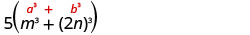Use the sum of cubes pattern.Simplify.Check. To check, you may find it easier to multiply the sum of cubes factors first, then multiply that product by 5. We’ll leave the multiplication for you.
5$$(m+2 n)\left(m^{2}-2 m n+4 n^{2}\right)$$
##### Exercise $$\PageIndex{49}$$

Factor: $$500 p^{3}+4 q^{3}$$

4$$(5 p+q)\left(25 p^{2}-5 p q+q^{2}\right)$$

##### Exercise $$\PageIndex{50}$$

Factor: $$432 c^{3}+686 d^{3}$$

2$$(6 c+7 d)\left(36 c^{2}-42 c d+49 d^{2}\right)$$

##### Note

Access these online resources for additional instruction and practice with factoring special products.

## Key Concepts

• Factor perfect square trinomials See Example. $$\begin{array} {lcc} \textbf { Step 1} \text { . Does the trinomial fit the pattern? } & a^{2}+2 a b+b^{2} & a^{2}-2 a b+b^{2} \\ \qquad \bullet \text { Is the first term a perfect square? } & (a)^{2} & (a)^{2} \\ \qquad \quad\text { Write it as a square. } \\ \qquad \bullet \text { Is the last term a perfect square? } & (a)^{2} \qquad\quad (b)^{2} & (a)^{2} \qquad \quad(b)^{2} \\ \qquad \quad \text { Write it as a square. } \\ \qquad \bullet \text { Check the middle term. Is it } 2 a b ? & (a)^{2} \searrow_{2 \cdot a \cdot b }\swarrow(b)^{2} & (a)^{2} \searrow_{2 \cdot a \cdot b} \swarrow(b)^{2} \\ \textbf { Step 2} . \text { Write the square of the binomial. } & (a+b)^{2} & (a-b)^{2} \\ \textbf { Step 3} . \text { Check by multiplying. }\end{array}$$
• Factor differences of squares See Example. $$\begin{array}{lc} \textbf { Step 1} . \text { Does the binomial fit the pattern? } & a^{2}-b^{2} \\ \qquad \bullet \text { Is this a difference? } & \underline{\quad} - \underline{\quad} \\ \qquad \bullet \text { Are the first and last terms perfect squares? } \\ \textbf { Step 2} . \text { Write them as squares. } & (a)^{2}-(b)^{2} \\ \textbf { Step 3.} \text{ Write the product of conjugates. } & (a-b)(a+b) \\ \textbf { Step 4.} \text{ Check by multiplying. } \end{array}$$
• Factor sum and difference of cubes To factor the sum or difference of cubes: See Example.
1. Does the binomial fit the sum or difference of cubes pattern? Is it a sum or difference? Are the first and last terms perfect cubes?
2. Write them as cubes.
3. Use either the sum or difference of cubes pattern.
4. Simplify inside the parentheses
5. Check by multiplying the factors.

## Glossary

perfect square trinomials pattern
If a and b are real numbers,

$\begin{array}{cc} {a^2+2ab+b^2=(a+b)^2}&{a^2−2ab+b^2=(a−b)^2}\\ \nonumber \end{array}$

difference of squares pattern
If a and b are real numbers,sum and difference of cubes pattern

$\begin{array}{cc} {a^3+b^3=(a+b)(a^2−ab+b^2)}&{a^3−b^3=(a−b)(a^2+ab+b^2)}\\ \nonumber \end{array}$

7.4: Factor Special Products is shared under a CC BY-NC-SA license and was authored, remixed, and/or curated by OpenStax.